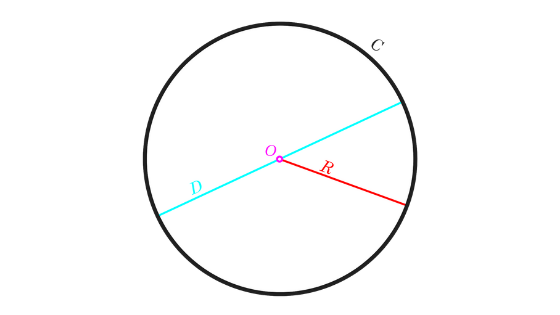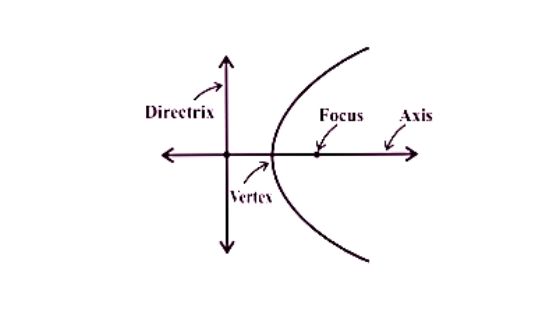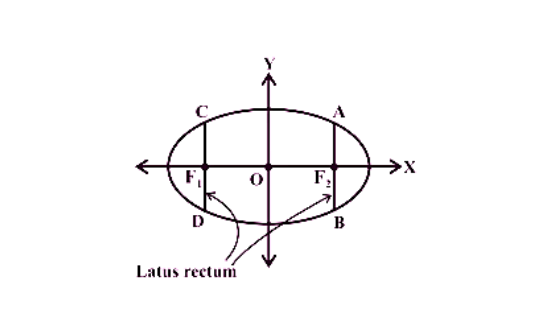#Also read, Circle Formulas Circle Theorems Lines and Angles Straight Line Coordinate Geometry

# Conic Section Formulas:

Since we have read simple geometrical figures in earlier classes. We already know about the importance of geometry in mathematics. Here we will learn conic section formulas. Circles, ellipses, parabolas and hyperbolas are in fact, known as conic sections or more commonly conics. As they can be obtained as intersections of any plane with a double-napped right circular cone. In fields such as planetary motion, design of telescopes and antennas, reflectors in flashlights and automobile headlights, etc. these curves have a very wide range of applications.

## Circle:

To know more about circle visit Circle Formula.

Standard Equation of Circle:
x2 + y2 = r2
Where r is radius of circle.## Parabola:

It’s interesting to know that (‘Para’ means ‘for’ and ‘bola’ means ‘throwing’, i.e., the shape described when you throw a ball in the air). Conic section formulas for the parabola is listed below.Equation of Parabola:

 S. No. Standard equation of Parabola Focus Directrix Parametric equation of parabola 1 y2 = 4ax (a, 0) x = -a x =at2 y =2at 2 y2 = – 4ax (-a, 0) x = a 3 x2 = 4ay (0, a) y = a 4 x2 = – 4ay (0, -a) y = – a
• Latus rectum of parabola: 4a

## Ellipse:

The set of every point in a plane, the sum of whose distances from two fixed points in the plane is a constant. Conic section formulas for Ellipse is listed below.Equation of Ellipse:

 S. No. Standard Equation of Ellipse Parametric Equation of ellipse F1 & F2 1 $$\frac{x^{2}}{a^{2}}$$ + $$\frac{y^{2}}{b^{2}}$$ = 1 x = a cos t y= b sin t \begin{aligned} & \text{if}~a \geq b \Longrightarrow F_1\left(-\sqrt{a^2-b^2},0\right)~~ F_2\left(\sqrt{a^2-b^2},0\right) \\ & \text{if}~a < b \Longrightarrow F_1\left(0, -\sqrt{b^2-a^2}\right) ~~ F_2\left(0, \sqrt{b^2-a^2}\right) \end{aligned} 2 $$\frac{x^{2}}{b^{2}}$$ + $$\frac{y^{2}}{a^{2}}$$ = 1
• Eccentricity of ellipse (e) = $$\frac{c}{a}$$ = $$\frac{\sqrt{a^2-b^2}}{a}$$
• Latus rectum of ellipse (l) = $$\frac{b^{2}}{a}$$
• Area of Ellipse = π⋅a⋅b

## Hyperbola:

The full set of all points in a plane, the difference of whose distances from two fixed points in the plane is a constant is Hyperbola. Conic section formulas for hyperbola is listed below.Equation of Hyperbola:

 S. No. Standard equation of Hyperbola Parametric equations of the Hyperbola: F1 & F2 1 $$\frac{x^{2}}{a^{2}}$$ – $$\frac{y^{2}}{b^{2}}$$ = 1 x = $$\frac{a}{sin\:t}$$ y = $$\frac{b\:sin\:t}{cos\:t}$$ \begin{aligned} & \text{if}~a \geq b \Longrightarrow F_1\left(-\sqrt{a^2+b^2},0\right)~~ F_2\left(\sqrt{a^2+b^2},0\right) \\ & \text{if}~a < b \Longrightarrow F_1\left(0, -\sqrt{a^2+b^2}\right) ~~ F_2\left(0, \sqrt{a^2+b^2}\right) \end{aligned} 2 $$\frac{y^{2}}{a^{2}}$$ – $$\frac{x^{2}}{b^{2}}$$ = 1
• Eccentricity of Hyperbola ( e ) = $$\frac{c}{a}$$
Also, c ≥ a, the eccentricity is never less than one.
• Distance of focus from centre: ae
• Equilateral hyperbola: Hyperbola in which a = b
• Conic section formulas for latus rectum in hyperbola: $$\frac{2b^{2}}{a}$$

## Conic section formulas examples:

1. Find an equation of the circle with centre at (0,0) and radius r.
Solution:
Here h = k = 0. Therefore, the equation of the circle is

x2 + y2= r2
2. Find the coordinates of the focus, axis, the equation of the directrix and latus rectum of the parabola y2 = 16x.
Solution:
In this equation, y2 is there, so the coefficient of x is positive so the parabola opens to the right. Comparing with the given equation y2 = 4ax, we find that a = 4.

Thus, the focus of the parabola is (4, 0) and the equation of the directrix of the parabola is x = – 4
Length of the latus rectum is 4a = 4 × 4 = 16.